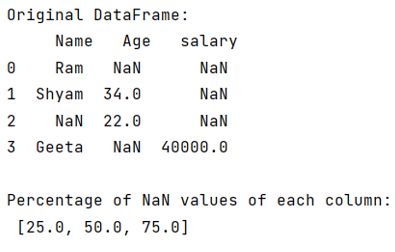# Python - Find out the percentage of missing values in each column in the given dataset

Learn, how to find out the percentage of missing values in each column in the given dataset?
Submitted by Pranit Sharma, on August 05, 2022

Pandas is a special tool that allows us to perform complex manipulations of data effectively and efficiently. Inside pandas, we mostly deal with a dataset in the form of DataFrame. DataFrames are 2-dimensional data structures in pandas. DataFrames consist of rows, columns, and data.

While creating a DataFrame or importing a CSV file, there could be some NaN values in the cells. NaN values mean "Not a Number" which generally means that there are some missing values in the cell. To deal with this type of data, you can either remove the particular row (if the number of missing values is low) or you can handle these values.

For handling these values, you might need to count the number of NaN values or you need to count the number of non-NaN values.

To find the percentage of NaN values in each column in the given dataset, we will first count the missing value in each column and apply the sum function.

After applying the sum function, we will divide this value by the length of the column so that we can get the percentage of NaN values.

Let us understand with the help of an example,

## Python code to find out the percentage of missing values in each column in the given dataset

```# Importing pandas package
import pandas as pd

# Importing numpy package
import numpy as np

# Creating a dictionary
d = {
'Name':["Ram","Shyam",np.NaN,"Geeta"],
'Age':[np.NaN,34,22,np.NaN],
'salary':[np.NaN,np.NaN,np.NaN,40000]
}

# Creating a DataFrame
df = pd.DataFrame(d)

# Display original DataFrame
print("Original DataFrame:\n",df,"\n")

# Calculating percentage
missing_values = []
result = df.count()
percentage = []

for i in range(len(result)):
missing_values.append(4-result[i])

for i in range(3):
percentage.append((missing_values[i]/4)*100)

# Display result
print("Percentage of NaN values of each column:\n",percentage)
```

Output:Languages: » C » C++ » C++ STL » Java » Data Structure » C#.Net » Android » Kotlin » SQL
Web Technologies: » PHP » Python » JavaScript » CSS » Ajax » Node.js » Web programming/HTML
Solved programs: » C » C++ » DS » Java » C#
Aptitude que. & ans.: » C » C++ » Java » DBMS
Interview que. & ans.: » C » Embedded C » Java » SEO » HR
CS Subjects: » CS Basics » O.S. » Networks » DBMS » Embedded Systems » Cloud Computing
» Machine learning » CS Organizations » Linux » DOS
More: » Articles » Puzzles » News/Updates